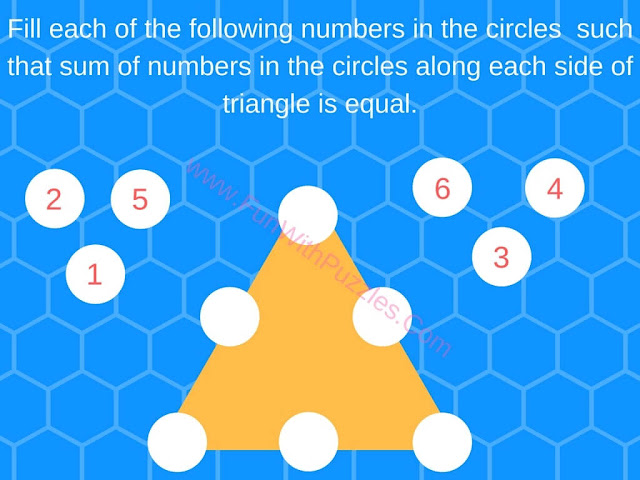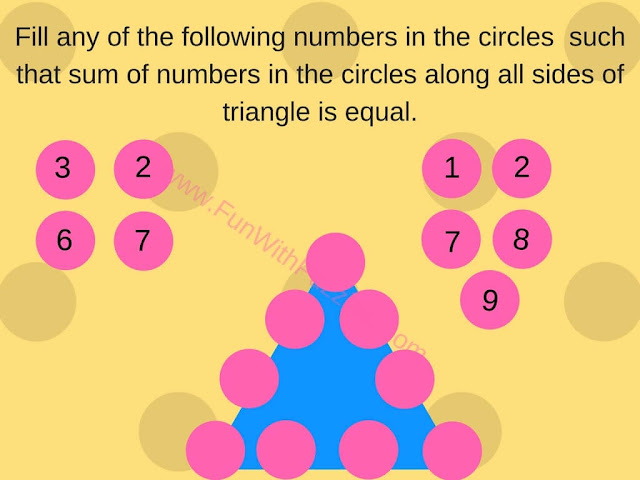Physical exercise is good for the body and mental exercise is good for the brain. So its time to do mental exercise with the quick mathematics brain test. This test is designed for teens. Kids may find it little tough and adults may find it easy. However, difficulty level depends upon one's experience in math and solving puzzles.
Here is a quick Mathematics brain test for teens. There are 4 mathematics puzzles. In each of the puzzles, some circles are given on the edges of the triangles. Corner circles are share between two edges. You are given numbers which you have to put in the circles so that the sum of numbers in the circles along each side of the triangle is equal. Do remember that you need to use given numbers exactly once.
Answers to these math brain puzzles will be given later at the end of this post. Do post your answers in the comment section of this post.1. Put given numbers in circles to make sum equal on each side.2. Put numbers in Circles to make sum on each side equal.3. Put given Numbers in Circles so that sum on each side of the triangle is the same.4. Put given numbers in circles to make each sum on sides the same.

Do check out some more Mathematical Brain Teasers to challenge your brain
List of Maths Puzzles and Brain Teasers

1. Fun Brain Teasers For Kids, Teens and Adults with Answers to Challenge your Mind: This contains not only Fun Maths Puzzles but also Logical and word brain teasers to tickle your mind.

2. Simple Math Brain Teasers to Twist your Brain: Here are simple yet mind-twisting brain teasers which is not only fun to solve but also will make you love Mathematics as a subject.

3. Math Circle Brain Teasers For Teens & Adults: This contains Maths Puzzles where numbers are put around the circle sharing some pattern. Your challenge is to find this common pattern and then solve for missing number.

4. Sudoku Variations: If you love solving the Maths Puzzles then you will definitely like solving printable Sudoku puzzles. Do check out different Sudoku Variations puzzles on this page.